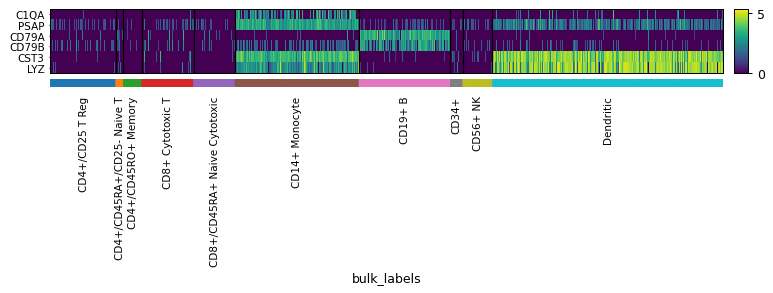# scanpy.pl.heatmap¶

`scanpy.pl.``heatmap`(adata, var_names, groupby, use_raw=None, log=False, num_categories=7, dendrogram=False, gene_symbols=None, var_group_positions=None, var_group_labels=None, var_group_rotation=None, layer=None, standard_scale=None, swap_axes=False, show_gene_labels=None, show=None, save=None, figsize=None, vmin=None, vmax=None, vcenter=None, norm=None, **kwds)Heatmap of the expression values of genes.

If `groupby` is given, the heatmap is ordered by the respective group. For example, a list of marker genes can be plotted, ordered by clustering. If the `groupby` observation annotation is not categorical the observation annotation is turned into a categorical by binning the data into the number specified in `num_categories`.

Parameters
adata : `AnnData``AnnData`

Annotated data matrix.

var_names :

`var_names` should be a valid subset of `adata.var_names`. If `var_names` is a mapping, then the key is used as label to group the values (see `var_group_labels`). The mapping values should be sequences of valid `adata.var_names`. In this case either coloring or ‘brackets’ are used for the grouping of var names depending on the plot. When `var_names` is a mapping, then the `var_group_labels` and `var_group_positions` are set.

groupby :

The key of the observation grouping to consider.

use_raw : (default: `None`)

Use `raw` attribute of `adata` if present.

log : `bool``bool` (default: `False`)

Plot on logarithmic axis.

num_categories : `int``int` (default: `7`)

Only used if groupby observation is not categorical. This value determines the number of groups into which the groupby observation should be subdivided.

categories_order

Order in which to show the categories. Note: add_dendrogram or add_totals can change the categories order.

figsize : (default: `None`)

Figure size when `multi_panel=True`. Otherwise the `rcParam['figure.figsize]` value is used. Format is (width, height)

dendrogram : (default: `False`)

If True or a valid dendrogram key, a dendrogram based on the hierarchical clustering between the `groupby` categories is added. The dendrogram information is computed using `scanpy.tl.dendrogram()`. If `tl.dendrogram` has not been called previously the function is called with default parameters.

gene_symbols : (default: `None`)

Column name in `.var` DataFrame that stores gene symbols. By default `var_names` refer to the index column of the `.var` DataFrame. Setting this option allows alternative names to be used.

var_group_positions : (default: `None`)

Use this parameter to highlight groups of `var_names`. This will draw a ‘bracket’ or a color block between the given start and end positions. If the parameter `var_group_labels` is set, the corresponding labels are added on top/left. E.g. `var_group_positions=[(4,10)]` will add a bracket between the fourth `var_name` and the tenth `var_name`. By giving more positions, more brackets/color blocks are drawn.

var_group_labels : (default: `None`)

Labels for each of the `var_group_positions` that want to be highlighted.

var_group_rotation : (default: `None`)

Label rotation degrees. By default, labels larger than 4 characters are rotated 90 degrees.

layer : (default: `None`)

Name of the AnnData object layer that wants to be plotted. By default adata.raw.X is plotted. If `use_raw=False` is set, then `adata.X` is plotted. If `layer` is set to a valid layer name, then the layer is plotted. `layer` takes precedence over `use_raw`.

standard_scale : {‘var’, ‘obs’} | `None``Optional`[`Literal`[‘var’, ‘obs’]] (default: `None`)

Whether or not to standardize that dimension between 0 and 1, meaning for each variable or observation, subtract the minimum and divide each by its maximum.

swap_axes : `bool``bool` (default: `False`)

By default, the x axis contains `var_names` (e.g. genes) and the y axis the `groupby` categories (if any). By setting `swap_axes` then x are the `groupby` categories and y the `var_names`.

show_gene_labels : (default: `None`)

By default gene labels are shown when there are 50 or less genes. Otherwise the labels are removed.

show : (default: `None`)

Show the plot, do not return axis.

save : (default: `None`)

If `True` or a `str`, save the figure. A string is appended to the default filename. Infer the filetype if ending on {`'.pdf'`, `'.png'`, `'.svg'`}.

ax

A matplotlib axes object. Only works if plotting a single component.

vmin : (default: `None`)

The value representing the lower limit of the color scale. Values smaller than vmin are plotted with the same color as vmin.

vmax : (default: `None`)

The value representing the upper limit of the color scale. Values larger than vmax are plotted with the same color as vmax.

vcenter : (default: `None`)

The value representing the center of the color scale. Useful for diverging colormaps.

norm : (default: `None`)

Custom color normalization object from matplotlib. See `https://matplotlib.org/stable/tutorials/colors/colormapnorms.html` for details.

**kwds

Are passed to `matplotlib.pyplot.imshow()`.

Returns

List of `Axes`

Examples

```import scanpy as sc